Скачать презентацию Chapter 7 Logic Sets and Counting Section 4

1498e64221ebe893b781a96e2981d50d.ppt

• Количество слайдов: 28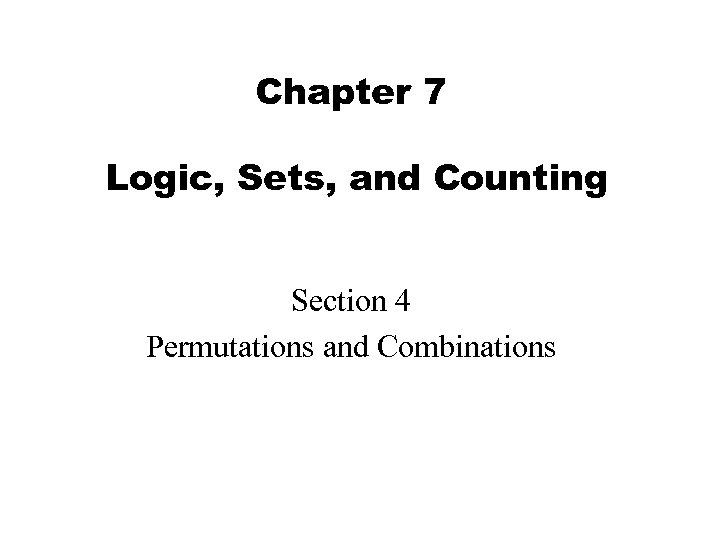Chapter 7 Logic, Sets, and Counting Section 4 Permutations and Combinations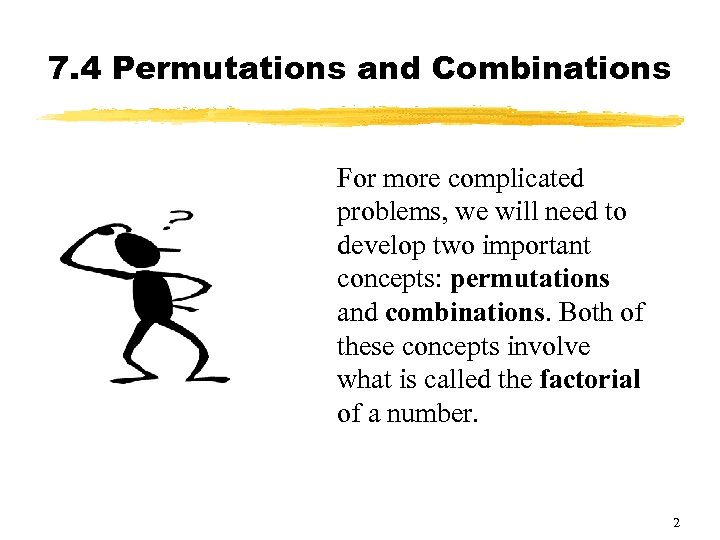7. 4 Permutations and Combinations For more complicated problems, we will need to develop two important concepts: permutations and combinations. Both of these concepts involve what is called the factorial of a number. 2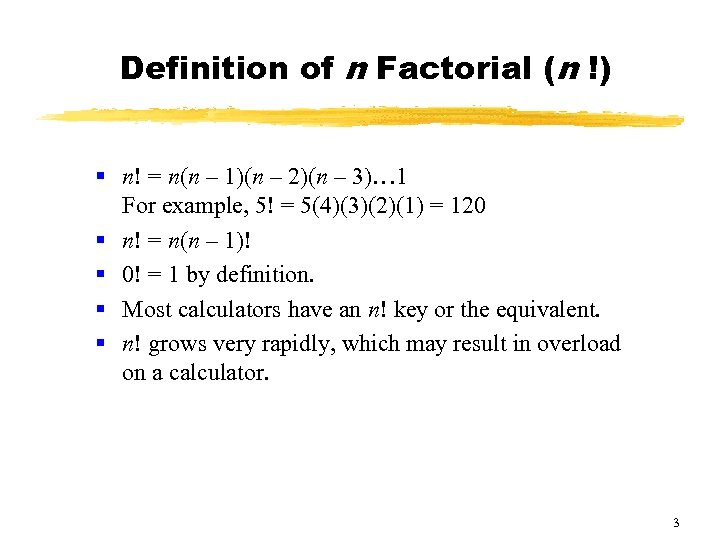Definition of n Factorial (n !) § n! = n(n – 1)(n – 2)(n – 3)… 1 For example, 5! = 5(4)(3)(2)(1) = 120 § n! = n(n – 1)! § 0! = 1 by definition. § Most calculators have an n! key or the equivalent. § n! grows very rapidly, which may result in overload on a calculator. 3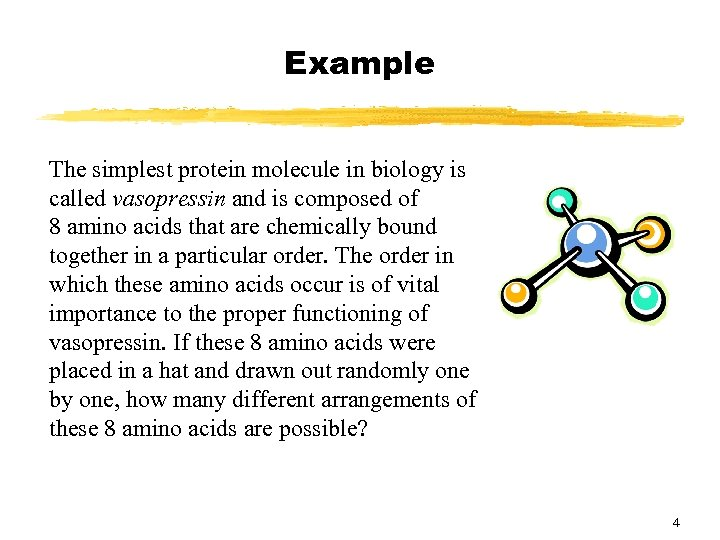Example The simplest protein molecule in biology is called vasopressin and is composed of 8 amino acids that are chemically bound together in a particular order. The order in which these amino acids occur is of vital importance to the proper functioning of vasopressin. If these 8 amino acids were placed in a hat and drawn out randomly one by one, how many different arrangements of these 8 amino acids are possible? 4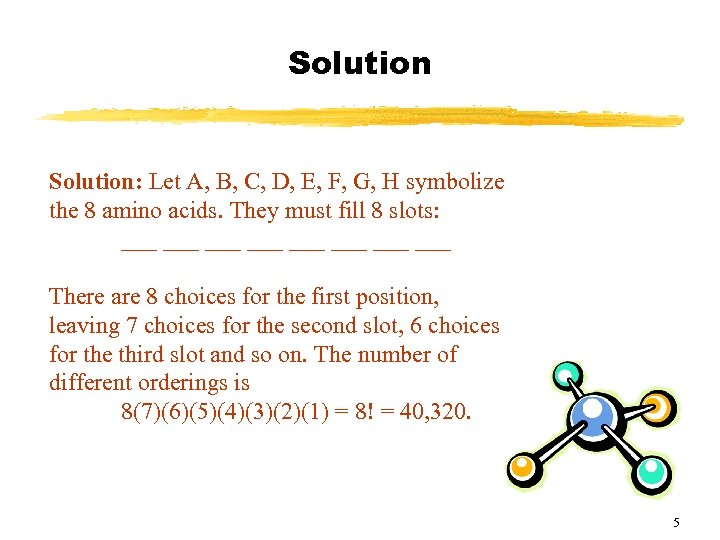Solution: Let A, B, C, D, E, F, G, H symbolize the 8 amino acids. They must fill 8 slots: ___ ___ There are 8 choices for the first position, leaving 7 choices for the second slot, 6 choices for the third slot and so on. The number of different orderings is 8(7)(6)(5)(4)(3)(2)(1) = 8! = 40, 320. 5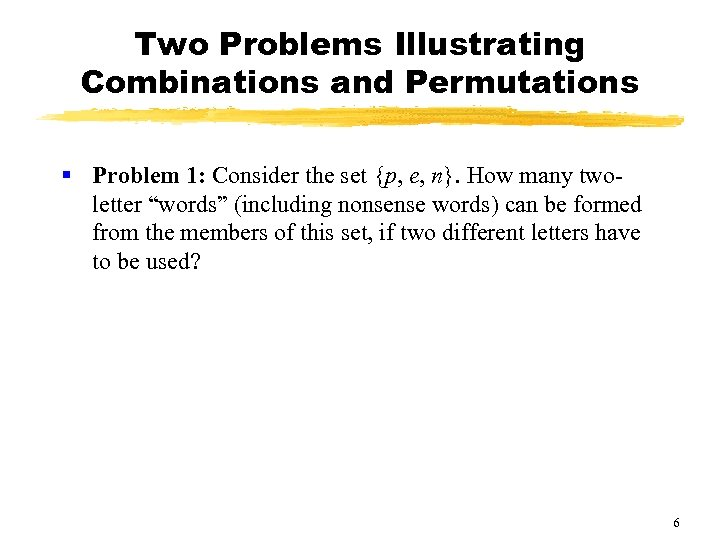Two Problems Illustrating Combinations and Permutations § Problem 1: Consider the set {p, e, n}. How many twoletter “words” (including nonsense words) can be formed from the members of this set, if two different letters have to be used? 6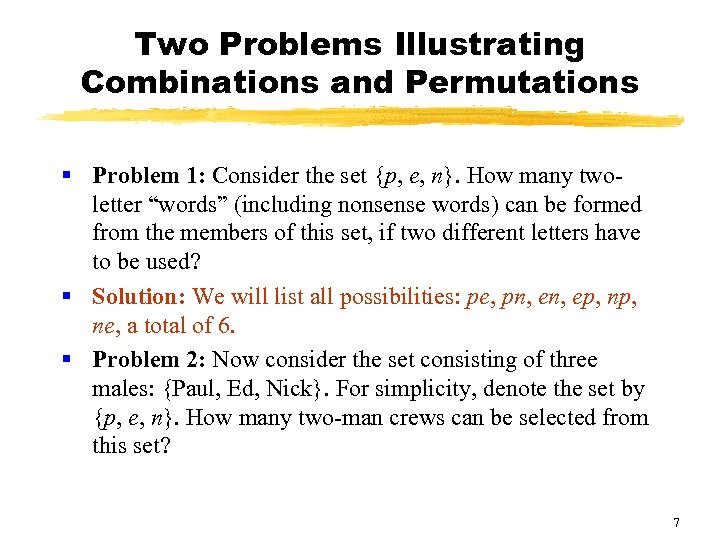Two Problems Illustrating Combinations and Permutations § Problem 1: Consider the set {p, e, n}. How many twoletter “words” (including nonsense words) can be formed from the members of this set, if two different letters have to be used? § Solution: We will list all possibilities: pe, pn, ep, ne, a total of 6. § Problem 2: Now consider the set consisting of three males: {Paul, Ed, Nick}. For simplicity, denote the set by {p, e, n}. How many two-man crews can be selected from this set? 7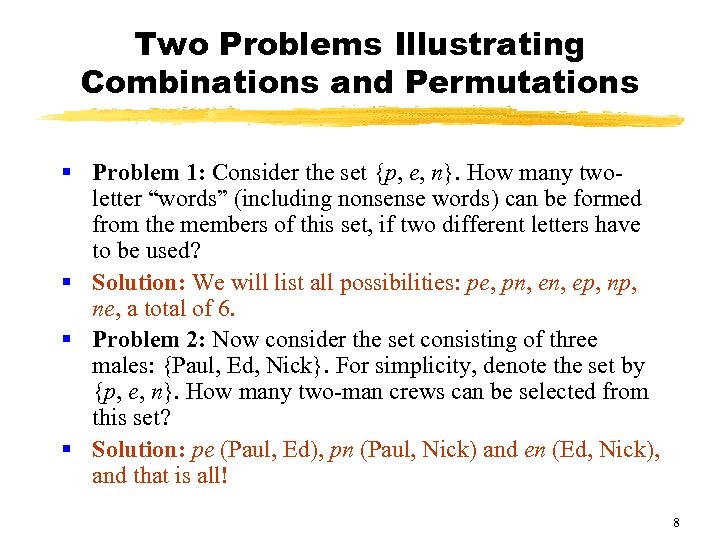Two Problems Illustrating Combinations and Permutations § Problem 1: Consider the set {p, e, n}. How many twoletter “words” (including nonsense words) can be formed from the members of this set, if two different letters have to be used? § Solution: We will list all possibilities: pe, pn, ep, ne, a total of 6. § Problem 2: Now consider the set consisting of three males: {Paul, Ed, Nick}. For simplicity, denote the set by {p, e, n}. How many two-man crews can be selected from this set? § Solution: pe (Paul, Ed), pn (Paul, Nick) and en (Ed, Nick), and that is all! 8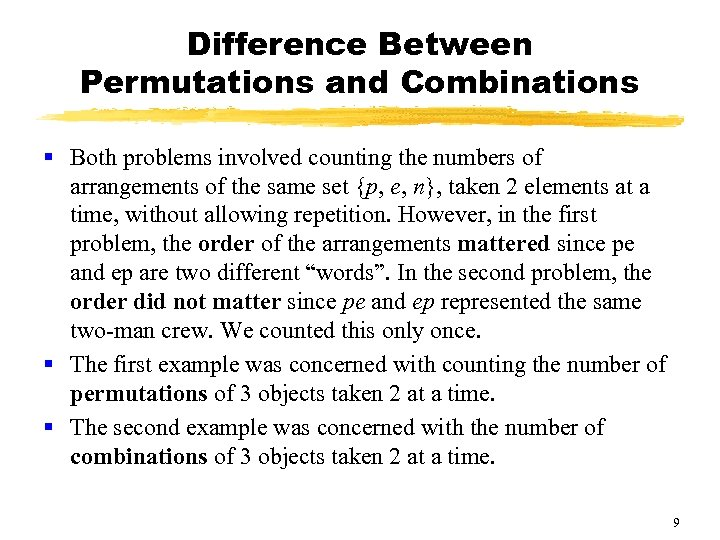Difference Between Permutations and Combinations § Both problems involved counting the numbers of arrangements of the same set {p, e, n}, taken 2 elements at a time, without allowing repetition. However, in the first problem, the order of the arrangements mattered since pe and ep are two different “words”. In the second problem, the order did not matter since pe and ep represented the same two-man crew. We counted this only once. § The first example was concerned with counting the number of permutations of 3 objects taken 2 at a time. § The second example was concerned with the number of combinations of 3 objects taken 2 at a time. 9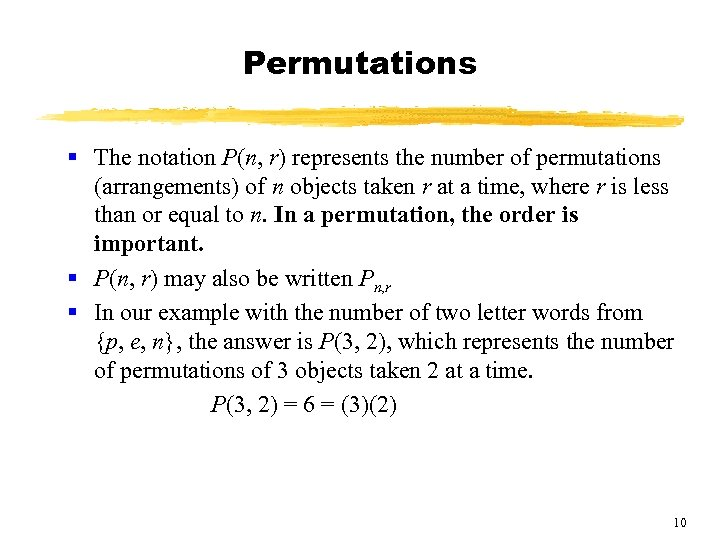Permutations § The notation P(n, r) represents the number of permutations (arrangements) of n objects taken r at a time, where r is less than or equal to n. In a permutation, the order is important. § P(n, r) may also be written Pn, r § In our example with the number of two letter words from {p, e, n}, the answer is P(3, 2), which represents the number of permutations of 3 objects taken 2 at a time. P(3, 2) = 6 = (3)(2) 10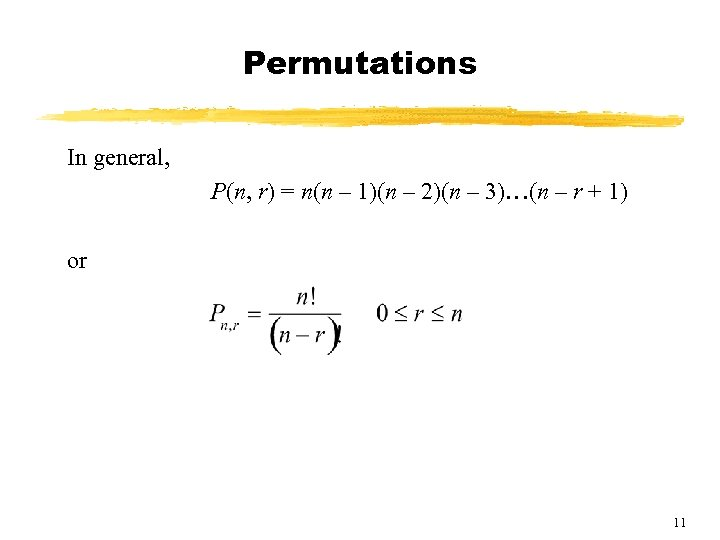Permutations In general, P(n, r) = n(n – 1)(n – 2)(n – 3)…(n – r + 1) or 11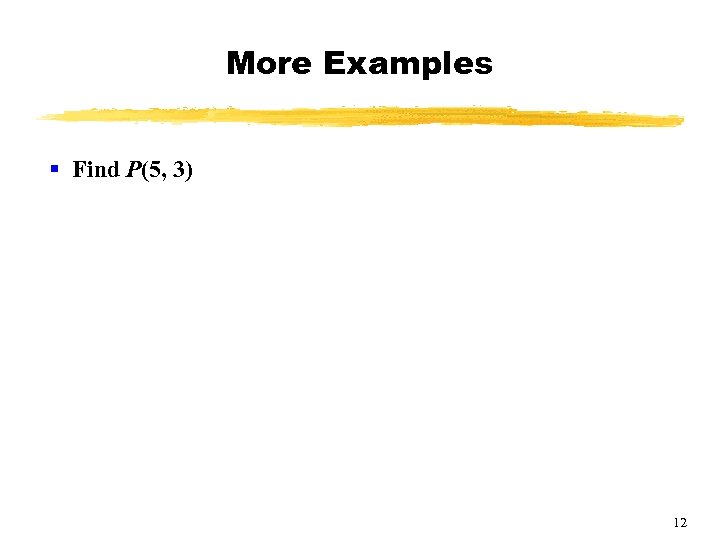More Examples § Find P(5, 3) 12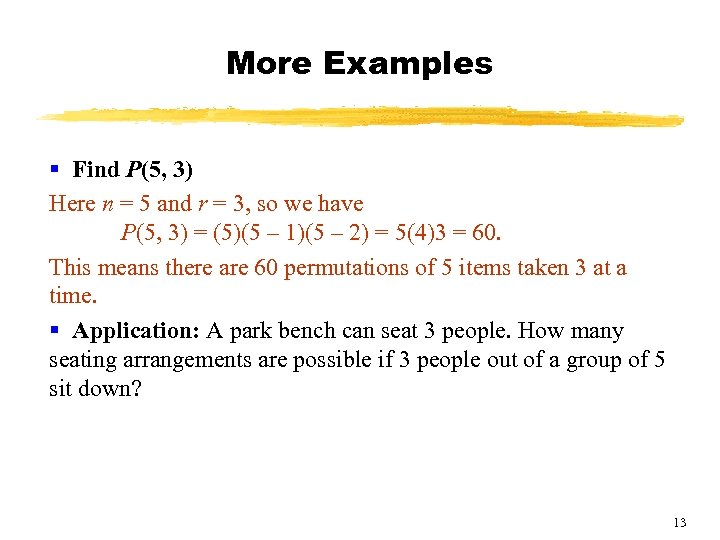More Examples § Find P(5, 3) Here n = 5 and r = 3, so we have P(5, 3) = (5)(5 – 1)(5 – 2) = 5(4)3 = 60. This means there are 60 permutations of 5 items taken 3 at a time. § Application: A park bench can seat 3 people. How many seating arrangements are possible if 3 people out of a group of 5 sit down? 13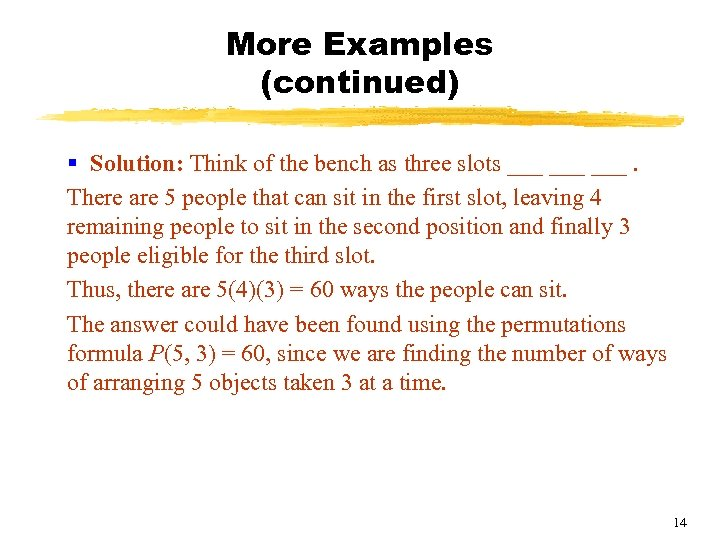More Examples (continued) § Solution: Think of the bench as three slots ___ ___. There are 5 people that can sit in the first slot, leaving 4 remaining people to sit in the second position and finally 3 people eligible for the third slot. Thus, there are 5(4)(3) = 60 ways the people can sit. The answer could have been found using the permutations formula P(5, 3) = 60, since we are finding the number of ways of arranging 5 objects taken 3 at a time. 14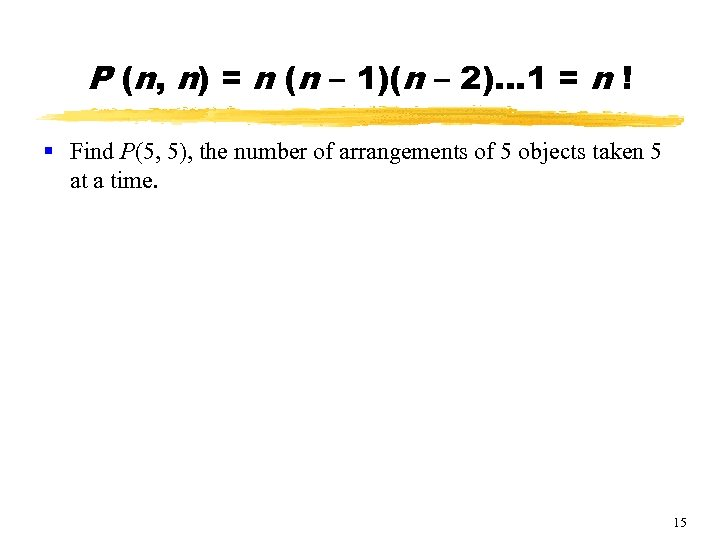P (n, n) = n (n – 1)(n – 2)… 1 = n ! § Find P(5, 5), the number of arrangements of 5 objects taken 5 at a time. 15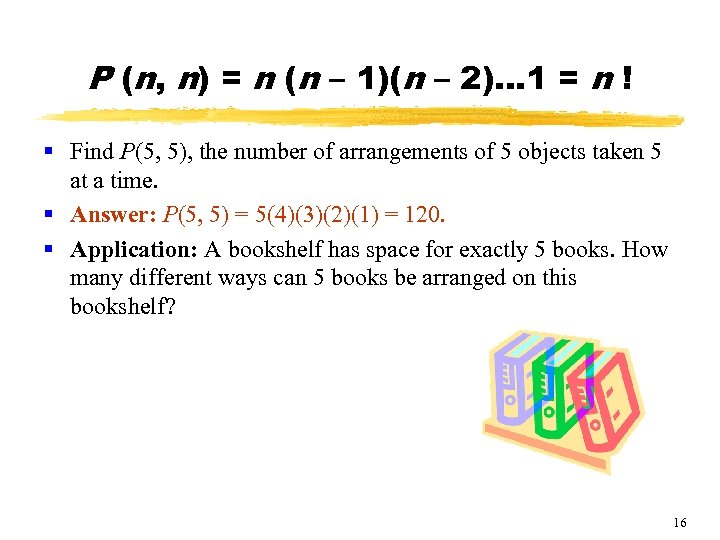P (n, n) = n (n – 1)(n – 2)… 1 = n ! § Find P(5, 5), the number of arrangements of 5 objects taken 5 at a time. § Answer: P(5, 5) = 5(4)(3)(2)(1) = 120. § Application: A bookshelf has space for exactly 5 books. How many different ways can 5 books be arranged on this bookshelf? 16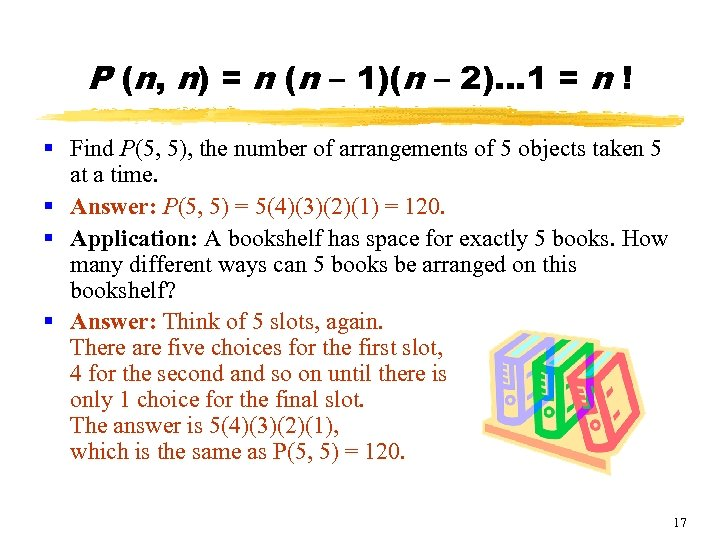P (n, n) = n (n – 1)(n – 2)… 1 = n ! § Find P(5, 5), the number of arrangements of 5 objects taken 5 at a time. § Answer: P(5, 5) = 5(4)(3)(2)(1) = 120. § Application: A bookshelf has space for exactly 5 books. How many different ways can 5 books be arranged on this bookshelf? § Answer: Think of 5 slots, again. There are five choices for the first slot, 4 for the second and so on until there is only 1 choice for the final slot. The answer is 5(4)(3)(2)(1), which is the same as P(5, 5) = 120. 17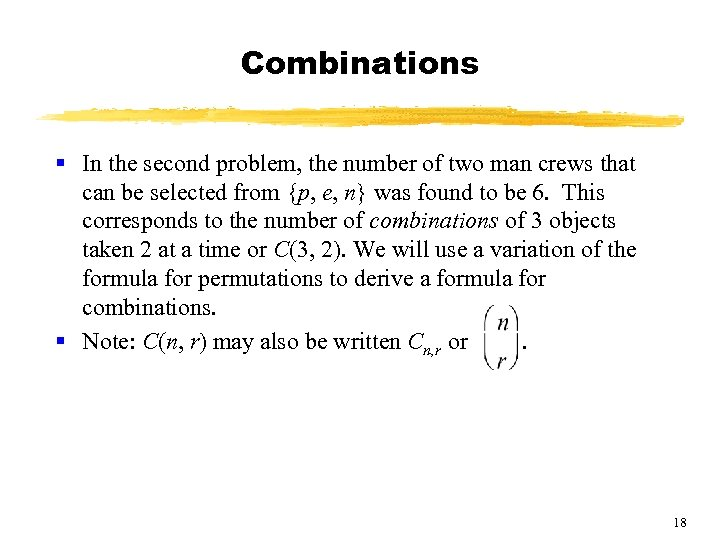Combinations § In the second problem, the number of two man crews that can be selected from {p, e, n} was found to be 6. This corresponds to the number of combinations of 3 objects taken 2 at a time or C(3, 2). We will use a variation of the formula for permutations to derive a formula for combinations. § Note: C(n, r) may also be written Cn, r or. 18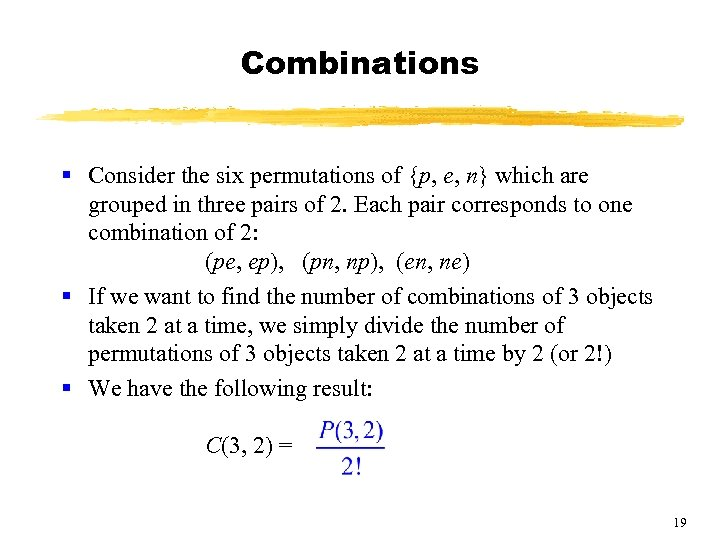Combinations § Consider the six permutations of {p, e, n} which are grouped in three pairs of 2. Each pair corresponds to one combination of 2: (pe, ep), (pn, np), (en, ne) § If we want to find the number of combinations of 3 objects taken 2 at a time, we simply divide the number of permutations of 3 objects taken 2 at a time by 2 (or 2!) § We have the following result: C(3, 2) = 19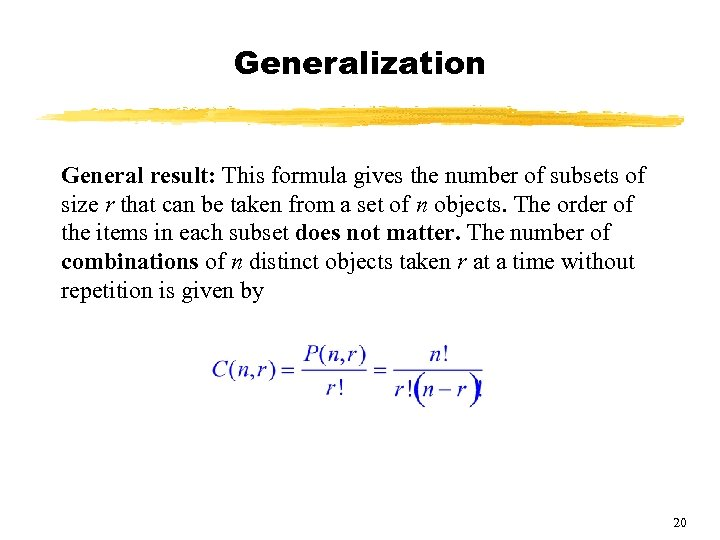Generalization General result: This formula gives the number of subsets of size r that can be taken from a set of n objects. The order of the items in each subset does not matter. The number of combinations of n distinct objects taken r at a time without repetition is given by 20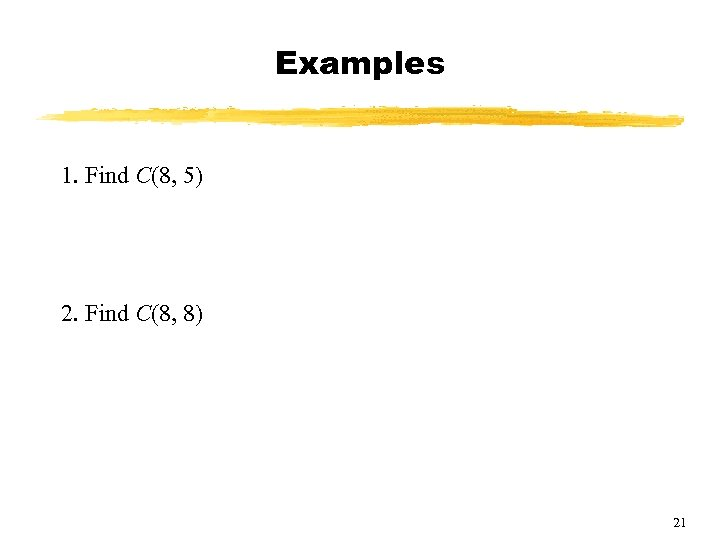Examples 1. Find C(8, 5) 2. Find C(8, 8) 21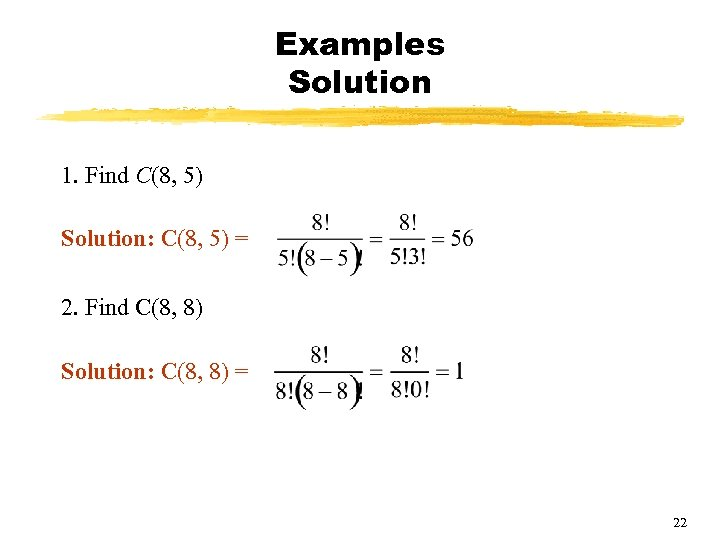Examples Solution 1. Find C(8, 5) Solution: C(8, 5) = 2. Find C(8, 8) Solution: C(8, 8) = 22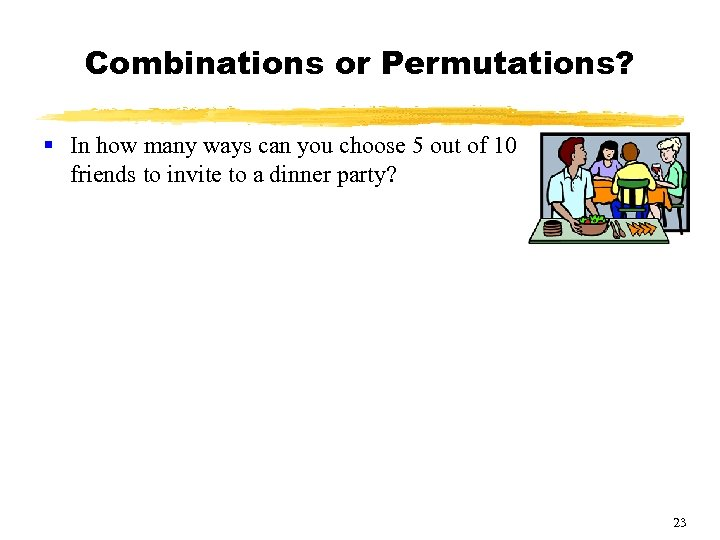Combinations or Permutations? § In how many ways can you choose 5 out of 10 friends to invite to a dinner party? 23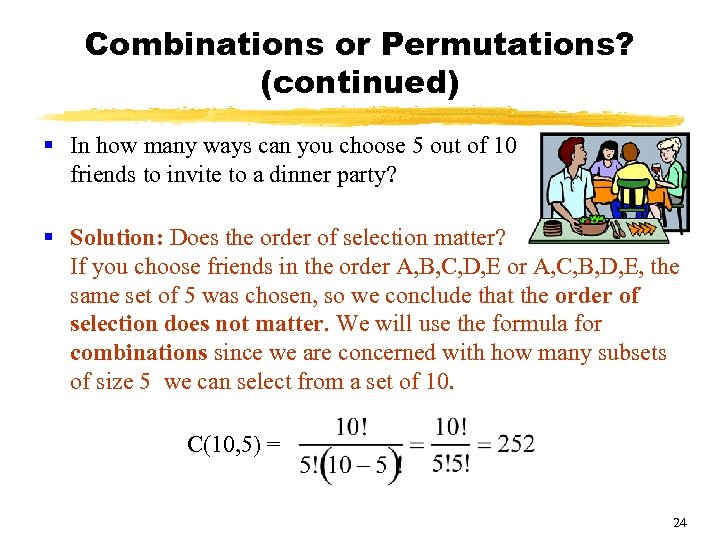Combinations or Permutations? (continued) § In how many ways can you choose 5 out of 10 friends to invite to a dinner party? § Solution: Does the order of selection matter? If you choose friends in the order A, B, C, D, E or A, C, B, D, E, the same set of 5 was chosen, so we conclude that the order of selection does not matter. We will use the formula for combinations since we are concerned with how many subsets of size 5 we can select from a set of 10. C(10, 5) = 24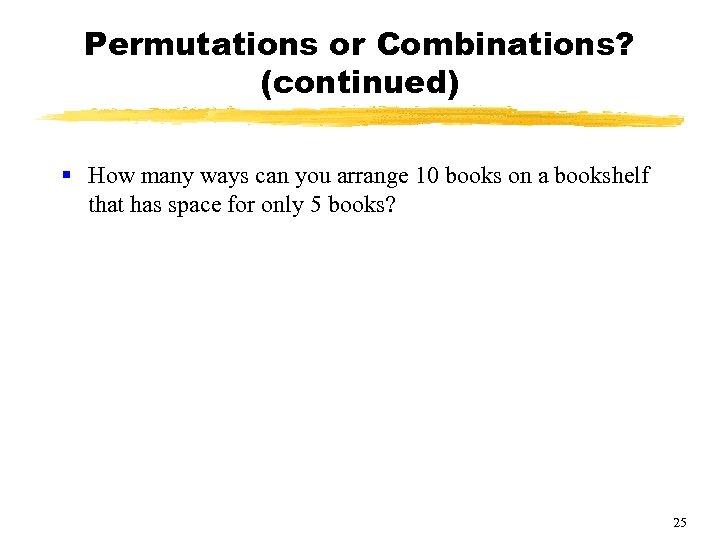Permutations or Combinations? (continued) § How many ways can you arrange 10 books on a bookshelf that has space for only 5 books? 25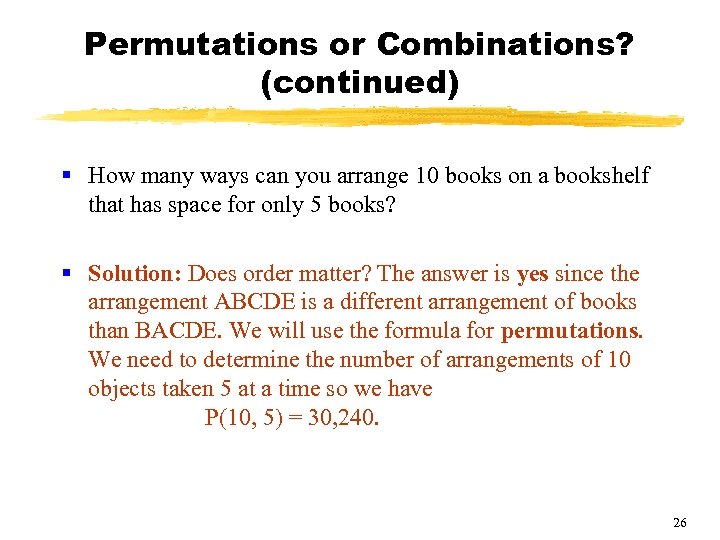Permutations or Combinations? (continued) § How many ways can you arrange 10 books on a bookshelf that has space for only 5 books? § Solution: Does order matter? The answer is yes since the arrangement ABCDE is a different arrangement of books than BACDE. We will use the formula for permutations. We need to determine the number of arrangements of 10 objects taken 5 at a time so we have P(10, 5) = 30, 240. 26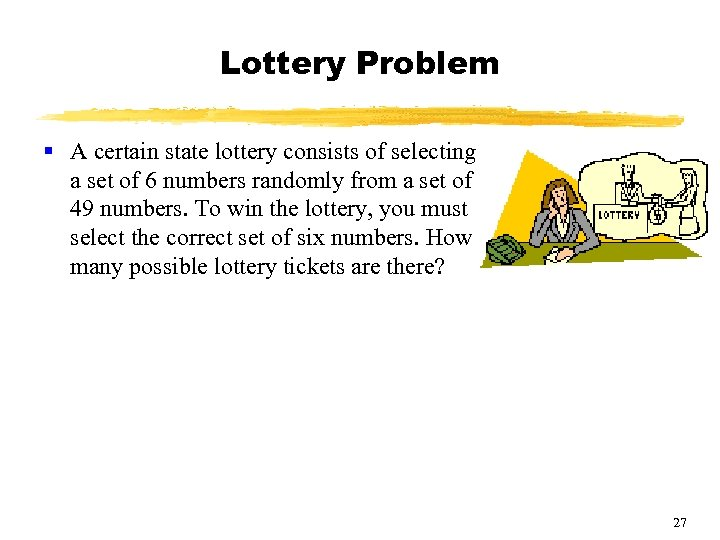Lottery Problem § A certain state lottery consists of selecting a set of 6 numbers randomly from a set of 49 numbers. To win the lottery, you must select the correct set of six numbers. How many possible lottery tickets are there? 27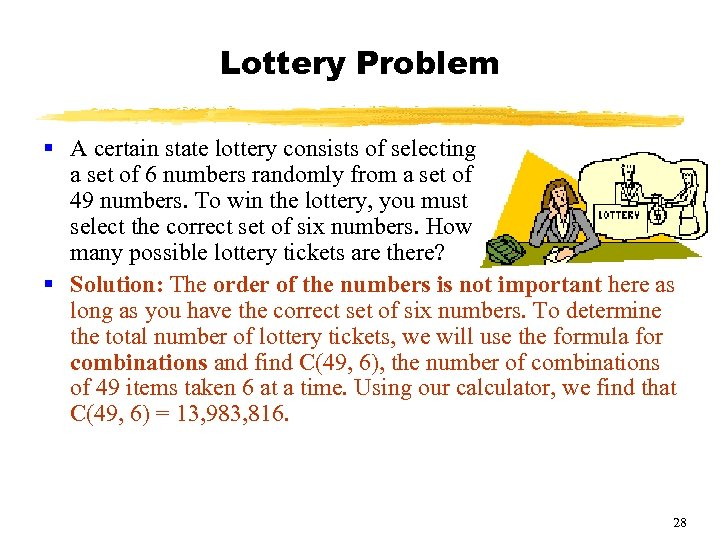Lottery Problem § A certain state lottery consists of selecting a set of 6 numbers randomly from a set of 49 numbers. To win the lottery, you must select the correct set of six numbers. How many possible lottery tickets are there? § Solution: The order of the numbers is not important here as long as you have the correct set of six numbers. To determine the total number of lottery tickets, we will use the formula for combinations and find C(49, 6), the number of combinations of 49 items taken 6 at a time. Using our calculator, we find that C(49, 6) = 13, 983, 816. 28